• +91 9971497814
• info@interviewmaterial.com

# Chapter 9 Areas of Parallelograms and Triangles Ex- 9.4 Interview Questions Answers

### Related Subjects

Question 1 :

Parallelogram ABCD and rectangle ABEF are on the same base AB and haveequal areas. Show that the perimeter of the parallelogram is greater than thatof the rectangle.

Answer 1 :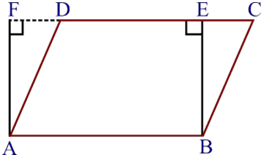Given,

|| gm ABCD and a rectangleABEF have the same base AB and equal areas.

To prove,

Perimeter of || gmABCD is greater than the perimeter of rectangle ABEF.

Proof,

We know that, theopposite sides of a|| gm and rectangle are equal.

, AB = DC [As ABCD isa || gm]

and, AB = EF [As ABEFis a rectangle]

, DC = EF … (i)

Adding AB on bothsides, we get,

AB + DC = AB + EF …(ii)

We know that, theperpendicular segment is the shortest of all the segments that can be drawn toa given line from a point not lying on it.

, BE < BC and AF< AD

BC > BE and AD> AF

AB+BC+CD+DA > AB+BE+EF+FA

perimeter of || gmABCD > perimeter of rectangle ABEF.

, the perimeter of theparallelogram is greater than that of the rectangle.

Hence Proved.

Question 2 :

In Fig. 9.30, D and E are two points on BC such that BD = DE = EC.

Show that ar (ABD) = ar (ADE) = ar (AEC).

Can you now answer the question that you have left in the ‘Introduction’of this chapter, whether the field of Budhia has been actually divided intothree parts of equal area?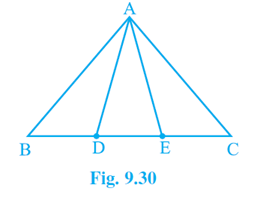Given,

BD = DE = EC

To prove,

ar (ABD) = ar (ADE) = ar (AEC)

Proof,

In (ABE), AD is median [since, BD = DE, given]

We know that, themedian of a triangle divides it into two parts of equal areas

, ar(ABD) = ar(AED) —(i)

Similarly,

In (ADC), AE is median [since, DE = EC, given]

From the equation (i)and (ii), we get

Question 3 :

In Fig. 9.31, ABCD, DCFE and ABFE are parallelograms. Show that ar(ADE) = ar (BCF).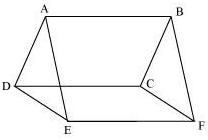Given,

ABCD, DCFE and ABFEare parallelograms

To prove,

Proof,

AD = BC [Since, theyare the opposite sides of the parallelogram ABCD]

DE = CF [Since, theyare the opposite sides of the parallelogram DCFE]

AE = BF [Since, theyare the opposite sides of the parallelogram ABFE]

, ADE BCF [Using SSS Congruence theorem]

, ar(ADE) = ar(BCF) [ By CPCT]

Question 4 :

In Fig. 9.32, ABCD is a parallelogram and BC is produced to a point Qsuch that AD = CQ. If AQ intersect DC at P, show that ar (BPC) = ar (DPQ).

[Hint : Join AC.]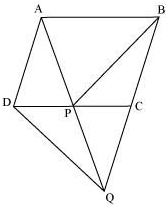Given:

ABCD is aparallelogram

To prove:

ar (BPC) = ar (DPQ)

Proof:

APD = QPC [Vertically Opposite Angles]

, ABO ACD [AAS congruency]

, DP = CP [CPCT]

In CDQ, QP is median. [Since, DP = CP]

Since, median of atriangle divides it into two parts of equal areas.

, ar(DPQ) = ar(QPC) —(i)

In PBQ, PC is median. [Since, AD = CQ and AD = BC BC = QC]

Since, median of atriangle divides it into two parts of equal areas.

, ar(QPC) = ar(BPC) —(ii)

From the equation (i)and (ii), we get

ar(BPC) = ar(DPQ)

Question 5 :

In Fig.9.33, ABC and BDE are two equilateral triangles such that D isthe mid-point of BC. If AE intersects BC at F, show that: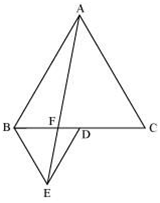(i) ar (BDE) =1/4 ar (ABC)

(ii) ar (BDE) = ½ ar (BAE)

(iii) ar (ABC) = 2 ar (BEC)

(iv) ar (BFE) = ar (AFD)

(v) ar (BFE) = 2 ar (FED)

(vi) ar (FED) = 1/8 ar (AFC)

(i) Assume that G andH are the mid-points of the sides AB and AC respectively.

Join the mid-pointswith line-segment GH. Here, GH is parallel to third side.

, BC will be half ofthe length of BC by mid-point theorem.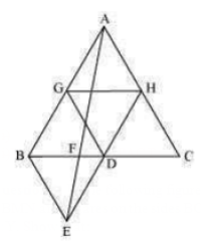GH =1/2 BC and GH ||BD

GH = BD = DC and GH|| BD (Since, D is the mid-point of BC)

Similarly,

GD = HC = HA

HD = AG = BG

, ΔABC is divided into4 equal equilateral triangles ΔBGD, ΔAGH, ΔDHC and ΔGHD

We can say that,

ΔBGD = ¼ ΔABC

Considering, ΔBDG andΔBDE

BD = BD (Common base)

Since both trianglesare equilateral triangle, we can say that,

BG = BE

DG = DE

, ΔBDG ΔBDE [By SSScongruency]

, area (ΔBDG) = area(ΔBDE)

ar (ΔBDE) = ¼ ar(ΔABC)

Hence proved

(ii)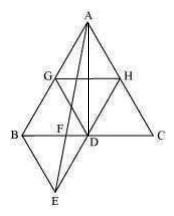ar(ΔBDE) = ar(ΔAED)(Common base DE and DE||AB)

ar(ΔBDE)−ar(ΔFED) =ar(ΔAED)−ar (ΔFED)

ar(ΔBEF) = ar(ΔAFD)…(i)

Now,

ar(ΔABD) =ar(ΔABF)+ar(ΔAFD)

ar(ΔABD) =ar(ΔABF)+ar(ΔBEF) [From equation (i)]

ar(ΔABD) = ar(ΔABE)…(ii)

ar(ΔABD) = ½ ar (ΔABC)

= (4/2) ar (ΔBDE)

= 2 ar (ΔBDE)…(iii)

From (ii) and (iii),we obtain

2 ar (ΔBDE) = ar(ΔABE)

ar (BDE) = ½ ar (BAE)

Hence proved

(iii) ar(ΔABE) =ar(ΔBEC) [Common base BE and BE || AC]

ar(ΔABF) + ar(ΔBEF) =ar(ΔBEC)

From eqn (i),we get,

ar(ΔABF) + ar(ΔAFD) =ar(ΔBEC)

ar(ΔABD) = ar(ΔBEC)

½ ar(ΔABC) = ar(ΔBEC)

ar(ΔABC) = 2 ar(ΔBEC)

Hence proved

(iv) ΔBDE and ΔAED lieon the same base (DE) and are in-between the parallel lines DE and AB.

ar (ΔBDE) = ar (ΔAED)

Subtracting ar(ΔFED)from L.H.S and R.H.S,

We get,

ar (ΔBDE)−ar (ΔFED) =ar (ΔAED)−ar (ΔFED)

ar (ΔBFE) = ar(ΔAFD)

Hence proved

(v) Assume that h isthe height of vertex E, corresponding to the side BD in ΔBDE.

Also assume that H isthe height of vertex A, corresponding to the side BC in ΔABC.

While solving Question(i),

We saw that,

ar (ΔBDE) = ¼ ar (ΔABC)

While solving Question(iv),

We saw that,

ar (ΔBFE) = ar (ΔAFD).

ar (ΔBFE) = ar (ΔAFD)

= 2 ar (ΔFED)

Hence, ar (ΔBFE) = 2ar (ΔFED)

Hence proved

(vi) ar (ΔAFC) = ar(ΔAFD) + ar(ΔADC)

= 2 ar (ΔFED) + (1/2)ar(ΔABC) [using (v)

= 2 ar (ΔFED) + ½[4ar(ΔBDE)] [Using result of Question (i)]

= 2 ar (ΔFED) +2ar(ΔBDE)

Since, ΔBDE and ΔAEDare on the same base and between same parallels

= 2 ar (ΔFED) +2 ar(ΔAED)

= 2 ar (ΔFED) +2 [ar(ΔAFD) +ar (ΔFED)]

= 2 ar (ΔFED) +2 ar(ΔAFD) +2 ar (ΔFED) [From question (viii)]

= 4 ar (ΔFED) +4 ar(ΔFED)

ar (ΔAFC) = 8 ar(ΔFED)

ar (ΔFED) = (1/8) ar(ΔAFC)

Hence proved

Question 6 :

6. Diagonals AC and BD of a quadrilateral ABCD intersect each other at P.Show that

ar (APB)×ar (CPD) = ar (APD)×ar (BPC).

Given:

The diagonal AC and BDof the quadrilateral ABCD, intersect each other at point E.

Construction:

From A, draw AMperpendicular to BD

From C, draw CNperpendicular to BD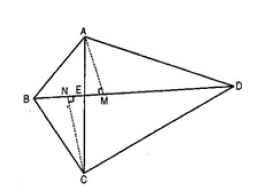To Prove,

ar(ΔAED) ar(ΔBEC) = ar(ΔABE) ×ar (ΔCDE)

Proof,

ar(ΔABE) = ½×BE×AM………….. (i)

ar(ΔAED) = ½×DE×AM………….. (ii)

Dividing eq. ii by i ,we get,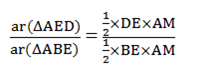ar(AED)/ar(ABE) =DE/BE…….. (iii)

Similarly,

ar(CDE)/ar(BEC) =DE/BE ……. (iv)

From eq. (iii) and(iv) , we get

ar(AED)/ar(ABE) =ar(CDE)/ar(BEC)

, ar(ΔAED)×ar(ΔBEC) =ar(ΔABE)×ar (ΔCDE)

Hence proved.

Question 7 :

7. P and Q are respectively the mid-points ofsides AB and BC of a triangle ABC and R is the mid-point of AP, show that:

(i) ar (PRQ) = ½ ar (ARC)

(ii) ar (RQC) = (3/8) ar (ABC)

(iii)ar (PBQ) = ar (ARC)

(i)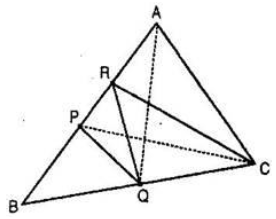We know that, mediandivides the triangle into two triangles of equal area,

PC is the median ofABC.

Ar (ΔBPC) = ar (ΔAPC)……….(i)

RC is the median ofAPC.

Ar (ΔARC) = ½ ar(ΔAPC) ……….(ii)

PQ is the median ofBPC.

Ar (ΔPQC) = ½ ar(ΔBPC) ……….(iii)

From eq. (i) and(iii), we get,

ar (ΔPQC) = ½ ar(ΔAPC) ……….(iv)

From eq. (ii) and(iv), we get,

ar (ΔPQC) = ar (ΔARC)……….(v)

P and Q are themid-points of AB and BC respectively [given]

PQ||AC

and, PA = ½ AC

Since, trianglesbetween same parallel are equal in area, we get,

ar (ΔAPQ) = ar (ΔPQC)……….(vi)

From eq. (v) and (vi),we obtain,

ar (ΔAPQ) = ar (ΔARC)……….(vii)

R is the mid-point ofAP.

, RQ is the median ofAPQ.

Ar (ΔPRQ) = ½ ar(ΔAPQ) ……….(viii)

From (vii) and (viii),we get,

ar (ΔPRQ) = ½ ar (ΔARC)

Hence Proved.

(ii) PQ is the medianof ΔBPC

ar (ΔPQC) = ½ ar(ΔBPC)

= (½) ×(1/2 )ar (ΔABC)

= ¼ ar (ΔABC) ……….(ix)

Also,

ar (ΔPRC) = ½ ar(ΔAPC) [From (iv)]

ar (ΔPRC) =(1/2)×(1/2)ar ( ABC)

= ¼ ar(ΔABC) ……….(x)

Add eq. (ix) and (x),we get,

ar (ΔPQC) + ar (ΔPRC)= (1/4)×(1/4)ar (ΔABC)

ar (quad. PQCR) = ¼ ar(ΔABC) ……….(xi)

Subtracting ar (ΔPRQ)from L.H.S and R.H.S,

ar (quad. PQCR)–ar(ΔPRQ) = ½ ar (ΔABC)–ar (ΔPRQ)

ar (ΔRQC) = ½ ar(ΔABC) – ½ ar (ΔARC) [From result (i)]

ar (ΔARC) = ½ ar(ΔABC) –(1/2)×(1/2)ar (ΔAPC)

ar (ΔRQC) = ½ ar(ΔABC) –(1/4)ar (ΔAPC)

ar (ΔRQC) = ½ ar(ΔABC) –(1/4)×(1/2)ar (ΔABC) [ As, PC is median of ΔABC]

ar (ΔRQC) = ½ ar(ΔABC)–(1/8)ar (ΔABC)

ar (ΔRQC) =[(1/2)-(1/8)]ar (ΔABC)

ar (ΔRQC) = (3/8)ar(ΔABC)

(iii) ar (ΔPRQ) = ½ ar(ΔARC) [From result (i)]

2ar (ΔPRQ) = ar (ΔARC)……………..(xii)

ar (ΔPRQ) = ½ ar(ΔAPQ) [RQ is the median of APQ] ……….(xiii)

But, we know that,

ar (ΔAPQ) = ar (ΔPQC)[From the reason mentioned in eq. (vi)] ……….(xiv)

From eq. (xiii) and(xiv), we get,

ar (ΔPRQ) = ½ ar(ΔPQC) ……….(xv)

At the same time,

ar (ΔBPQ) = ar (ΔPQC)[PQ is the median of ΔBPC] ……….(xvi)

From eq. (xv) and(xvi), we get,

ar (ΔPRQ) = ½ ar(ΔBPQ) ……….(xvii)

From eq. (xii) and(xvii), we get,

2×(1/2)ar(ΔBPQ)= ar(ΔARC)

ar (ΔBPQ) = ar (ΔARC)

Hence Proved.

Question 8 :

In Fig. 9.34, ABC is a right triangle right angled at A. BCED, ACFGand ABMN are squares on the sides BC, CA and AB respectively. Line segment AX ^DE meets BC at Y. Show that: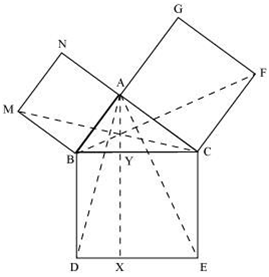(i) ΔMBC ΔABD

(ii) ar(BYXD) = 2ar(MBC)

(iii) ar(BYXD) = ar(ABMN)

(iv) ΔFCB ΔACE

(v) ar(CYXE) = 2ar(FCB)

(vi) ar(CYXE) = ar(ACFG)

(vii) ar(BCED) = ar(ABMN)+ar(ACFG)

Note: Result (vii) is the famous Theoremof Pythagoras. You shall learn a simpler proof of this theorem in class X.

(i) We know that eachangle of a square is 90°. Hence, ABM = DBC = 90º

∴∠ABM+ABC = DBC+ABC

∴∠MBC = ABD

In ∆MBC and ∆ABD,

MBC = ABD (Proved above)

MB = AB (Sides ofsquare ABMN)

BC = BD (Sides ofsquare BCED)

∆MBC ∆ABD (SAS congruency)

(ii) We have

∆MBC ∆ABD

ar (∆MBC) = ar (∆ABD)… (i)

It is given that AX DE and BD DE (Adjacent sides of square BDEC)

BD || AX (Two linesperpendicular to same line are parallel to each other)

∆ABD and parallelogramBYXD are on the same base BD and between the same parallels BD and AX.

Area (∆YXD) = 2 Area(∆MBC) [From equation (i)] … (ii)

(iii) ∆MBC andparallelogram ABMN are lying on the same base MB and between same parallels MBand NC.

2 ar (∆MBC) = ar(ABMN)

ar (∆YXD) = ar (ABMN)[From equation (ii)] … (iii)

(iv) We know that eachangle of a square is 90°.

∴∠FCA = BCE = 90º

∴∠FCA+ACB = BCE+ACB

∴∠FCB = ACE

In ∆FCB and ∆ACE,

FCB = ACE

FC = AC (Sides ofsquare ACFG)

CB = CE (Sides ofsquare BCED)

∆FCB ∆ACE (SAS congruency)

(v) AX DE and CE DE (Adjacent sides of square BDEC) [given]

Hence,

CE || AX (Two linesperpendicular to the same line are parallel to each other)

Consider BACE andparallelogram CYXE

BACE and parallelogramCYXE are on the same base CE and between the same parallels CE and AX.

ar (∆YXE) = 2ar (∆ACE)… (iv)

∆FCB ∆ACE

ar (∆FCB) ar (∆ACE) … (v)

From equations (iv)and (v), we get

ar (CYXE) = 2 ar(∆FCB) … (vi)

(vi) Consider BFCB andparallelogram ACFG

BFCB and parallelogramACFG are lying on the same base CF and between the same parallels CF and BG.

ar (ACFG) = 2 ar(∆FCB)

ar (ACFG) = ar (CYXE)[From equation (vi)] … (vii)

(vii) From the figure,we can observe that

ar (∆CED) = ar(∆YXD)+ar (CYXE)

ar (∆CED) = ar(ABMN)+ar (ACFG) [From equations (iii) and (vii)].

Todays Deals### Chapter 9 Areas of Parallelograms and Triangles Ex- 9.4 Contributorskrishan

Name:
Email:

# Latest News# 9000 interview questions in different categories